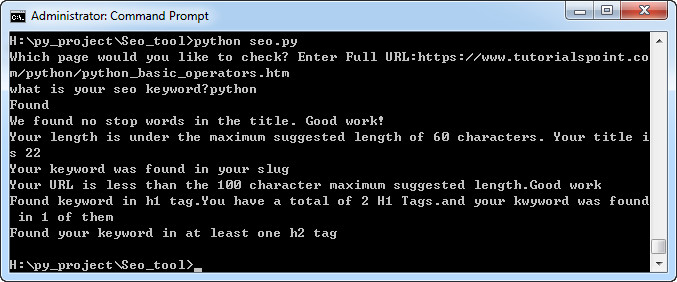# Python开发SEO工具

XAMPP教程 843浏览`1` `from urllib.request import urlopen,Request`
 `2` `from bs4 import BeautifulSoup`
 `3` `import re`
 `4`
 `5`
 `6` `url = input(``"Which page would you like to check? Enter Full URL:"``)`
 `7` `keyword = input(``"what is your seo keyword?"``)`
 `8` `keyword = keyword.casefold()`
 `9`
 `10` `try:`
 `11` `    ``req = Request(url, headers = {``'User-Agent'``:``'Mozilla/6.0'``})`
 `12` `    ``html = urlopen(req)`
 `13` `except HTTPError ``as` `e:`
 `14` `    ``print``(e)`
 `15`
 `16` `data = BeautifulSoup(html,``"html.parser"``)`
 `17`
 `18` `def seo_title_found(keyword,data):`
 `19` `    ``if` `data.title:`
 `20` `        ``if` `keyword in data.title.text.casefold():`
 `21` `            ``status = ``"Found"`
 `22` `        ``else``:`
 `23` `            ``status = ``"Not Found"`
 `24` `    ``else``:`
 `25` `        ``status = ``"No title found"`
 `26`
 `27` `    ``return` `status`
 `28`
 `29` `def seo_title_stop_words(data):`
 `30` `    ``words = 0`
 `31` `    ``list_words = []`
 `32` `    ``if` `data.title:`
 `33` `        ``with open(``'stopwords.txt'``,``'r'``) ``as` `f:`
 `34` `            ``for` `line in f:`
 `35` `                ``if` `re.search(r``'\b'` `+ line.rstrip(``'\n'``)+r``'\b'``,data.title.text.casefold()):`
 `36` `                    ``words += 1`
 `37` `                    ``list_words.append(line.rstrip(``'\n'``))`
 `38` `        ``if` `words > 0:`
 `39` `            ``stop_words = ``"We found {} stop words in your title. You should consider removing them.{}"``.format(words,list_words)`
 `40` `        ``else``:`
 `41` `            ``stop_words = ``"We found no stop words in the title. Good work!"`
 `42` `    ``else``:`
 `43` `        ``stop_words = ``"We could not find a title"`
 `44`
 `45` `    ``return` `stop_words`
 `46`
 `47`
 `48` `def seo_title_length(data):`
 `49` `    ``if` `data.title:`
 `50` `        ``if` `len(data.title.text) < 60:`
 `51` `            ``length = ``"Your length is under the maximum suggested length of 60 characters. Your title is {}"``.format(len(data.title.text))`
 `52` `        ``else``:`
 `53` `            ``length = ``"Your length is over the maximum suggest length of 60 character. Your title is {}"``.format(len(data.title.text))`
 `54` `    ``else``:`
 `55` `        ``length = ``"No title was found"`
 `56`
 `57` `    ``return` `length`
 `58`
 `59` `def se_url(url):`
 `60` `    ``if` `url:`
 `61` `        ``if` `keyword in url:`
 `62` `            ``slug = ``"Your keyword was found in your slug"`
 `63` `        ``else``:`
 `64` `            ``slug = ``"Your keywords was not found in your slug"`
 `65` `    ``else``:`
 `66` `        ``slug = ``"No url was retured"`
 `67` `    ``return` `slug`
 `68`
 `69` `def seo_url_length(url):`
 `70` `    ``if` `url:`
 `71` `        ``if` `len(url) < 100:`
 `72` `            ``url_length = ``"Your URL is less than the 100 character maximum suggested length.Good work"`
 `73` `        ``else``:`
 `74` `            ``url_length =  ``"Your URL is over 100 characters. Your URL currently is {}.You should change this."``.format(len(url))`
 `75` `    ``else``:`
 `76` `        ``url_length = ``"URL was not found"`
 `77` `    ``return`  `url_length`
 `78`
 `79` `def seo_h1(keyword, data):`
 `80` `    ``total_h1 = 0`
 `81` `    ``total_keyword_h1 = 0`
 `82` `    ``if` `data.h1:`
 `83` `        ``all_tags = data.find_all(``'h1'``)`
 `84` `        ``for` `tag in all_tags:`
 `85` `            ``total_h1 += 1`
 `86` `            ``tag = str(tag.string)`
 `87` `            ``if` `keyword in tag.casefold():`
 `88` `                ``total_keyword_h1 += 1`
 `89` `                ``h1_tag = ``"Found keyword in h1 tag.You have a total of {} H1 Tags.and your kwyword was found in {} of them"``.format(total_h1,total_keyword_h1)`
 `90` `            ``else``:`
 `91` `                ``h1_tag = ``"Didn't find a kwyords in h1 tag."`
 `92` `    ``else``:`
 `93` `        ``h1_tag = ``"No H1 Tags Found"`
 `94` `    ``return` `h1_tag`
 `95`
 `96` `def seo_h2(keyword,daa):`
 `97` `    ``if` `data.h2:`
 `98` `        ``all_tags = data.find_all(``'h2'``)`
 `99` `        ``for` `tag in all_tags:`
 `100` `            ``tag = str(tag.string)`
 `101` `            ``if` `keyword in tag.casefold():`
 `102` `                ``h2_tag = ``"Found your keyword in at least one h2 tag"`
 `103` `            ``else``:`
 `104` `                ``h2_tag = ``"We didn't find your keyword in a singel h2 tag.You should add {} to h2 tag"``.format(keyword)`
 `105` `    ``else``:`
 `106` `        ``h2_tag = ``"No h2 tags found.You  should have at least one containing your keyword."`
 `107` `    ``return` `h2_tag`
 `108`
 `109` `print``(seo_title_found(keyword,data))`
 `110` `print``(seo_title_stop_words(data))`
 `111` `print``(seo_title_length(data))`
 `112` `print``(se_url(url))`
 `113` `print``(seo_url_length(url))`
 `114` `print``(seo_h1(keyword,data))`
 `115` `print``(seo_h2(keyword,data))`